Next: Statistical Thermodynamics Up: Heat and Work Previous: Exact and Inexact Differentials

# Exercises

1. The mean pressure,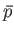, of a thermally insulated gas varies with volume according to the relation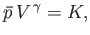where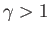and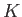are positive constants. Show that the work done by this gas in a quasi-static process in which the state of the gas evolves from an initial macrostate with pressure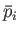and volume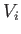to a final macrostate with pressure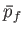and volume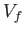is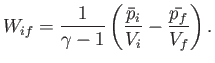2. Consider the infinitesimal quantity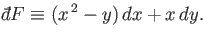Is this an exact differential? If not, find the integrating factor that converts it into an exact differential.

3. A system undergoes a quasi-static process that appears as a closed curve in a diagram of mean pressure,, versus volume,. Such a process is termed cyclic, because the system ends up in a final macrostate that is identical to its initial macrostate. Show that the work done by the system is given by the area contained within the closed curve in the-plane.Next: Statistical Thermodynamics Up: Heat and Work Previous: Exact and Inexact Differentials
Richard Fitzpatrick 2016-01-25